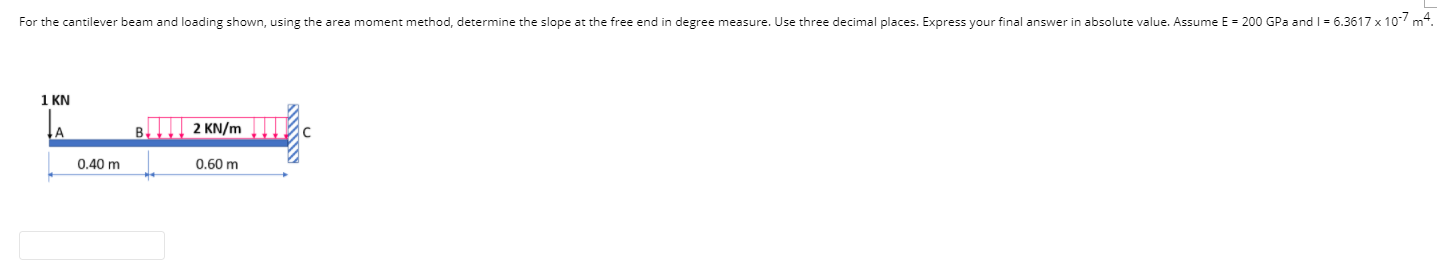# Question For the cantilever beam and loading shown, using the area moment method, determine the slope at the free end in degree measure. Use three decimal places. Express your final answer in absolute value. Assume E = 200 GPa and I = 6.3617x 10-7m4. 1 KN TA III, 2 KN/m B с 0.40 m 0.60 mBM1T9O The Asker · Civil EngineeringTranscribed Image Text: For the cantilever beam and loading shown, using the area moment method, determine the slope at the free end in degree measure. Use three decimal places. Express your final answer in absolute value. Assume E = 200 GPa and I = 6.3617x 10-7m4. 1 KN TA III, 2 KN/m B с 0.40 m 0.60 m
More
Transcribed Image Text: For the cantilever beam and loading shown, using the area moment method, determine the slope at the free end in degree measure. Use three decimal places. Express your final answer in absolute value. Assume E = 200 GPa and I = 6.3617x 10-7m4. 1 KN TA III, 2 KN/m B с 0.40 m 0.60 m